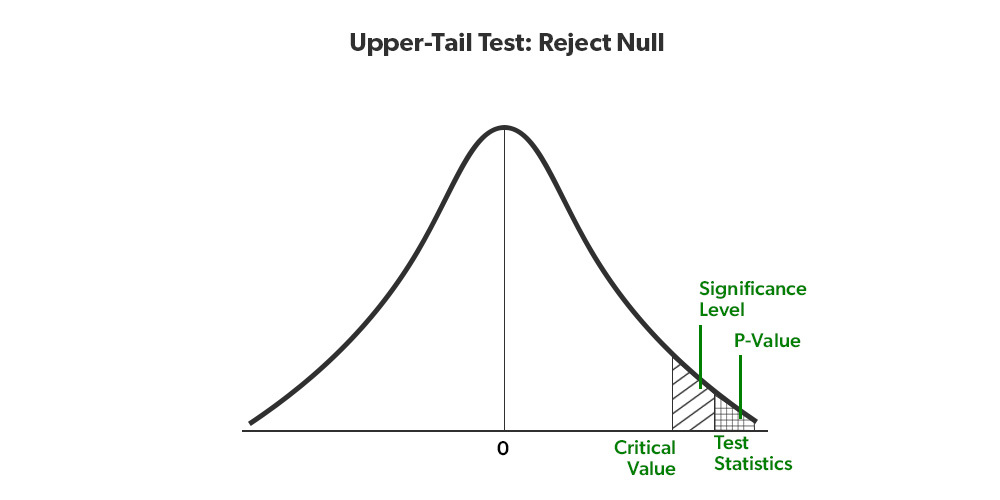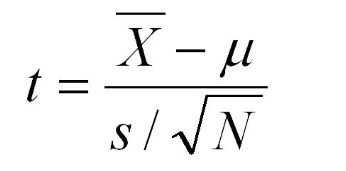# Upper Tail Test of Population Mean with Unknown Variance in R

• Last Updated : 02 Jun, 2022

A statistical hypothesis test is a method of statistical inference used to decide whether the data at hand sufficiently support a particular hypothesis. The conventional steps that are followed while formulating the hypothesis test, are listed as follows

• State null hypothesis (Ho) and alternate hypothesis (Ha)
• Collect a relevant sample of data to test the hypothesis.
• Choose significance level for the hypothesis test.
• Perform an appropriate statistical test.
• Based on test statistics and p-value decide whether to reject or fail to reject your null hypothesis.Conventionally, in an upper-tail test, the null hypothesis states that the true population mean (μo) is lesser than the hypothesized mean value (μ). We fail to reject the null hypothesis if the test statistic is lesser than the critical value at the chosen significance level. In this article let us discuss how to conduct an upper-tail test of the population mean with unknown variance.

Here the assumption is the population variance σ2  is unknown. Let s2 be the sample variance. For larger n (usually >30), the population of the following statistics of all possible samples of size n is approximately a Student t distribution with n – 1 degree of freedom (DOF).

Let us define the test statistic based on t-distribution as followsif t >= −tα, where tα is the 100(1 − α) percentile of the Student t distribution with n − 1 degree of freedom, we can reject the null hypothesis.

Let us try to understand the upper tail test with unknown variance by considering a case study.

Assume the data labeling company states that there are less than 2 errors in marked labels on any single page. Assume the actual mean amount of error per page 2.12, and the sample standard deviation is 0.2. At the .05 significance level, is it possible to reject the company claim for a sample size of 40 pages?

Null Hypothesis: Label errors per page <= 2

Alternate Hypothesis: Label errors per page > 2

Significance level: 0.05

Example:

Let us compute the test statistic

## R

 `xbar = 2 ``# sample mean ``mu0 = 2.12 ``# hypothesized value ``s = 0.2 ``# sample standard deviation ``n = 40 ``# sample size ``t = (xbar-mu0)/(s/``sqrt``(n)) ``t ``# test statistic`

Output:

`3.7947331`

Now, let us compute the critical value at a 0.05 significance level,

## R

 `alpha = .05 ``t.alpha = ``qt``(1-alpha, df=n-1) ``t.alpha ``# critical value`

Output:

`1.68487`

The test statistic 3.794733 is much greater than the critical value of 1.68487, which means according to our initial assumption, here t > tα , so we reject the null hypothesis.

Hence, at the .05 significance level, we reject the statement of the company that they mean labeling errors per page are not less than 2 for a sample of 40 pages. Here, we don’t have enough evidence to fail to reject the company’s claim.

My Personal Notes arrow_drop_up